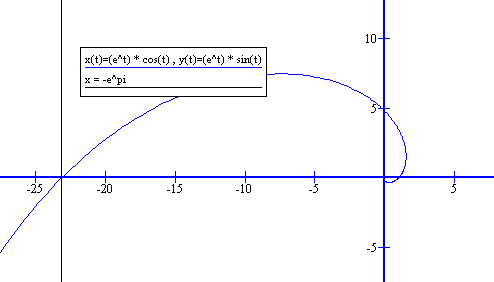1. ##Curvature

r(t) = < e^t * cost, e^t*sint, 5>
Find the curvature at the point (x, y, z) = (-e^π, 0, 5)
explain briefly how you come up with answer and also express answer as a decimal.

2. ## Re: Curvature

If a point $\displaystyle T(x,y,z)$ belongs to a curve $\displaystyle \vec{r}(t)=x(t)\vec{i}+y(t)\vec{j}+z(t)\vec{k}$ then exists a parameter value $\displaystyle t\in \mathbb{R}$ such that
$\displaystyle x=x(t), \, y=y(t), \, z=z(t)$. In case of a given point $\displaystyle t=-\pi.$

Wikipedia says that for a space curve its curvature is given by.

All derivatives are with respect to variable t. Here $\displaystyle \kappa$ is a function in variable $\displaystyle t$, $\displaystyle \kappa=\kappa (t).$ Find the expression for $\displaystyle \kappa(t)$ and evaluate it for $\displaystyle t=-\pi$.

3. ## Re: Curvature

Also, notice that as z is constant you are really looking at,... so you only need the 2-D version (Curvature - Wikipedia, the free encyclopedia).

Just in case a picture helps differentiate...... where (key in spoiler) ...

Spoiler:... is the product rule. Straight continuous lines differentiate downwards (integrate up) with respect to t. And,

_________________________________________

Don't integrate - balloontegrate!

Balloon Calculus; standard integrals, derivatives and methods

Balloon Calculus Drawing with LaTeX and Asymptote!

#### Search Tags

curvature